Pure and Applied Mathematics Journal
Volume 4, Issue 5-1, October 2015, Pages: 51-54

Optimization and Analysis on Binary Tree Selection Sort Algorithm

Wang Min, Li Yaolong

College of Media Engineering, Weinan Normal University, Weinan, Shanxi, P. R. China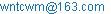(Wang Min)

Wang Min, Li Yaolong. Optimization and Analysis on Binary Tree Selection Sort Algorithm. Pure and Applied Mathematics Journal. Special Issue: Mathematical Aspects of Engineering Disciplines. Vol. 4, No. 5-1, 2015, pp. 51-54. doi: 10.11648/j.pamj.s.2015040501.20

Abstract: With giving the design ideas and the algorithm descriptions in C, the binary tree selection sort algorithm is analyzed and introduced in detail. After the time complexity and the space complexity of the algorithms being analyzed in this paper, the binary tree selection sort algorithm is compared with the simple selection sort algorithm. This paper provides a theoretical basis on algorithm optimization.

Keywords: Binary Tree Selection Sort, Time Complexity, Space Complexity

1. Introduction

Data structure and Algorithms are important and basic subjects in computer science, and they are an indivisible whole. The procedure of computer solving problem follow such steps that inputting the initial data, processing by the computer, and then outputting the information. For a given problem, it is essential to decide how to organize the initial data, how to store them in the computer, and how to choose and design appropriate algorithms for it. To solve a particular problem, it needs to analyze the problem at first, and then combine organically the data structure and algorithms. To solve the problem effectively, algorithms must adopt suitable data structures (see [1,2]).

Search operations are often needed in computer data processing. In order to use more efficient search method, it is often needed that the data to be processed in an ordered arrangement according to the keywords. Thus sort operation is one of the basic operations in computer programming (see [3,4]).

An n records sequence {R1, R2,…,Rn}, whoes corresponding sequence of keywords is {K1, K2,...,Kn}, is required to be sorted to identify a permutation p1, p2,..., pn of the current subscript sequence 1,2,...,n, so that the appropriate keywords meet the non-decreasing (or non-increasing) relationship, that is Kp1Kp2≤…≤Kpn, in order to get an ordered record sequence by their keywords. Such process is known as sort (see [3,4,5,6]).

As the number of records to be sorted is different, which makes the memories involved in sort processes are different, the sort process can be divided into two categories: One is the internal sort, referring to the process during which the records to be sorted are stored in the computer random access memory; the other is the external sort, referring to the process during which the external memories are still needed to be visited for the number of records to be sorted is too large for the memory to accommodate to (see [4,5,7,8]).

The basic design idea of selection sort algorithm is as follows: In each trip, select the minimum record keyword from the record keywords (total n-i+1, i=1,2,…,n-1) of the unordered table as the i-th record of the ordered sequence (see[3,7,9]). In simple selection sort, it needs n-1 comparisons to select the minimum from n record keywords, and n-2 comparisons to select the minimum from n-1 record keywords, and obviously, each trip doesn’t use the previous comparative result. Thus the time complexity of comparisons is O (n2). To reduce the number of comparisons, the comparison results need to be kept down during the comparing process (see ).

Most of the Chinese references about data structure are not detailed introduce on binary tree selection sort algorithm. This paper will focus on analyzing the binary tree selection sort algorithm design idea, giving the algorithm description in C, analyzing the algorithm time complexity and space complexity, and summarizing the advantages and disadvantages in comparison with the simple selection sort algorithm, so as to providing a theoretical basis for the traditional simple selection sort algorithm optimization.

2. Binary Tree Selection Sort Algorithm

The sort methods used by the sequence of records can be divided into three kinds: vector sort, table (or list) sort and address sort, according to the different storage ways. This paper chooses the first method, that is, a set of records to be sorted are stored in the address of a contiguous group of memory cells. The following assumes that all record keywords will be sorted in accordance with the non-decreasing order.

The type of records to be sorted can be defined in C as follows:

typedef struct{

KeyType key;

OtherType otherinfo;

} RecordType (see );

2.1. Analysis on Algorithm Design Ideas

Binary tree selection sort is known as tournament selection sort, and its basic design idea is as follows:

1.  Compare pair wise adjacent records in the records sequence, and select all smaller record keywords. The last record will be selected by itself if number n is odd;

2.  Select each smaller one of the two adjacent records selected from step 1;

3.  Repeat step 2 until the minimum record keyword is selected.

This process can be shown with a binary tree which has n leaf nodes, that is the amount of records for sorting (see ). The relevant descriptions mentioned that the selection tree is a complete binary tree (see [3,7,11]), but the actual may be not the same. When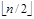is odd, for example, the corresponding binary tree, as shown in Fig. 1 with 6 record keywords, is obviously not a complete binary tree.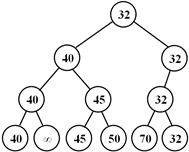Fig. 1. The corresponding binary tree of selecting the minimum keyword.

Although the selection tree may be not a complete binary tree, they are similar in shape, so the selection binary tree can also use the sequential storage structure for complete binary tree. According to the nature 4 of complete binary tree, the depth of the binary tree is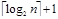when there are n records(the amount of leaf nodes) to be sorted, thus the storage units amount is the same as that of the nodes (total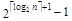) of full binary tree with the same depth. The amount of nodes before the records to be sorted is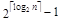according to nature 2 of complete binary tree. To meet the sequential storage definition of complete binary tree, we agreed that the serial number of the starting unit is 1. Therefore, the records to be sorted can be stored into the consecutive n units beginning at serial number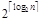(note that≥n) during initialization. After that, we can compare every two records (serial number i and i+1 records, where i=~+n-2) to select the smaller, and store it into the parent node location (serial number is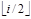) of the two records according to the nature 5 of complete binary tree.

In order to select the next minimum after outputting the first one, the following steps are described in ,  and  (see [3,7,11]):

1.  Set the leaf node of the minimum to be ∞;

2.  Select each smaller one of the two adjacent records selected from step 1; Re-compare from the leaf node set to be ∞, modify in turn each node in the path from the leaf node to the root node, and then the root node will be the next smallest keyword record (see Figure 2).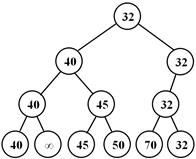Fig. 2. The binary tree of selecting the next minimum keyword process.

To implement the above procedure, the leaf node of the minimum keyword must be located at first; this can be realized by comparing the keyword of the root node with that of each leaf node. Set the minimum keyword to be ∞ after the corresponding leaf node was located. And then, judge the leaf node is the left child or right child of its parent, in order to determine which sibling to compare with to modify the value of its parent. Similarly, determine the parent node whose serial number is greater than 1 to compare with its left or right sibling to modify the value of its parent…Repeat this procedure until the root node, the next minimum keyword will be obtained.

2.2. Algorithm Description in C

Suppose the records to be sorted have already been stored in the consecutive n units from serial numberto+n-1 of the vector r, the binary tree selection sort algorithm can be described below in C based on the defined type RecordType (where n is the number of records to be sorted):

//Find the minimum.

void FindMinimum(RecordType r[], int n)

{

int i,j,m,h; //h is the depth of the tree.

h=Intlog2(n)+1; //Intlog2(n) returns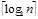.

m=(int)pow(2,h-1); // pow() returns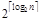.

//m is the beginning unit of the records to be sorted.

while(m>1)

{

for(i=m; i<m+n-1; i+=2)

{

j=i/2;

r[j]=r[i].key<=r[i+1].key?r[i]:r[i+1];

}//End_for

if(i==m+n-1) //n is odd.

r[i/2]=r[i];

n=n%2?n/2+1:n/2;

m/=2;

} //End_while

}//End

#define INFINITY 32767

//Find the next minimum among the rest.

void FindNextMinimum(RecordType r[],int n)

{

int i,m,h;

h=Intlog2(n)+1; //The definition is the same as before.

m=(int)pow(2,h-1);

for(i=m; i<=m+n-1&&r[i].key!=r.key; i++);

//Locate the minimum.

r [i].key=INFINITY;

while (i>1)

{

if((i-m+1)%2) //r[i] is the left child.

if(i==m+n-1||r[i].key<=r[i+1].key)

r [i/2]=r[i]; //r[i]is the last node of the level or

//its keyword is smaller than that of its right sibling.

else

r [i/2]=r[i+1]; // End_if

else

if (r [i].key<=r[i-1]. key)

r[i/2]=r[i];

else

r[i/2]=r[i-1]; //End_if

i/=2;

n=n%2?n/2+1:n/2;

m/=2;

}//End_while

}//End

3. Algorithm Testing and Evaluation

To verify the correctness of the functions FindMinimum() and FindNextMinimum(), a logarithm function Intlog2(), an initialization function Initialization(), an output function Print() and the calling function main() were added to ensure the integrity of the programs, so as to test them in Visual C ++ 6.0 environment. Function Intlog2() returns the base 2 logarithmic value of the up rounded; function Initialization() store the records to be sorted into the serial number from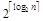to+n-1 of the vector r; function Print() outputs sequentially subscript 1~n units of all record keywords; function main() includes initializing records vector, calling function FindMinimum(), cyclically calling function FindNextMinimum(), and outputting the record keywords after each sorting trip and so on. Where the sentence segment of cyclically calling function FindNextMinimum() was as follows:

for(i=1;i<n;i++)

{

rs[i]=r;

FindNextMinimum(r,n);

...

}

Meanwhile, the Key Type was set to be int, the RecordType remained only the keyword member key for simplifying the input and the output, and the infinity value ∞ was set to be 32767. Fig.3 shows the running result samples of the algorithm program in VC ++ 6.0 environment.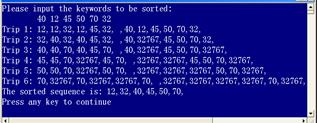Fig. 3. Example of the running result of the binary selection sort algorithm in VC ++ 6.0 environment.

Regard the records number n as the problem scale. From the algorithm description in C of the binary tree selection sort algorithm, you can see that the core statements in function FindMinimum() is the nested double loop. The loop body of the surrounding loop (while loop) executestimes, which equals to the depth of the binary selection tree; while the loop body of the surrounded loop (for loop) executes n/2 times, which depends on the number of records to be sorted. Therefore, the executing times of the basic statements in loop body is n(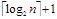)/2, and the average time complexity of this function is T(n)=O(n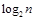). In addition to the vector space used by the record elements, the function also doubled the auxiliary vector space and introduced variables i, j, m and h, so its space complexity is linear order, that is S(n)=O(n).

The core statements in function FindNextMinimum() is consists of one for loop and one while loop executed sequentially. The for loop locates the minimum keyword, and the comparing times is n; the while loop sets the minimum to be ∞, and modifies the parents in each level, so the executing times of the basic statements in loop body is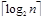. Therefore, the total executing times of the basic statements in this function is nearly n+. Since the function call in the calling function main() is nested in a for loop, and the calling times is n, the executing times of the basic statements in loop body is n(n+), and therefore the average time complexity of this function is T(n)=O(n2+n)=O(n2). Similarly, this function also doubled the auxiliary vector space and introduced variables i, m and h, so its space complexity is also linear order, that is S(n)=O(n).

4. Conclusion

After analyzing the time complexity and space complexity of the binary tree selection sort algorithm, we can draw the following conclusions ultimately:

Compared with the simple selection sort algorithm, the binary tree selection sort algorithm introduced more auxiliary spaces, and therefore made the space complexity increased. This algorithm needs to locate the minimum during the procedure of selecting the next minimum, so its time efficiency is nearly the same as that of the simple selection sort algorithm. Obviously, this point is probably overlooked in ,  and  (see [3,7,12]).

This paper aims to go deep into the analysis on binary tree selection sort algorithm, so as to giving atheoretical basic for the traditional simple selection sort algorithm optimization, and playing a guiding role in teaching the relevant chapters in "Data Structure" curriculum.

Acknowledgements

This work was conducted partially with financial support from the scientific research program (No. 2014JM1026) funded by Shaanxi Science and Technology Agency, and with scientific research program (No. 13YKS015) funded by Weinan Normal University.

References

1. Wang Weidong, Data Structure Learning guidance. Xi'an Electronic Science and Technology University Press, Xi'an (2004).
2. Min Wang and Yunfei Li, "Designing on a Special Algorithm of Triple Tree Based on the Analysis of Data Structure", International Conference on Computer Education, Simulation and Modeling(CESM 2011), Proceedings, Part I(Communications in Computer and Information Science), 423-427.
3. Geng Guohua, Data Structure—C Language Description, Xi'an: Xi'an Electronic Science and Technology University Press, China, (2006), 228-241.
4. Wang Min, "Analysis on 2-Element Insertion Sort Algorithm", 2010 International Conference on Computer Design and Applications(ICCDA 2010),Volume 1,Session 1-C: Computer Theory, 143-145.
5. Xu Xiaokai. Simple Data Structure Tutorial. Tsinghua University Press, Beijing, (1995),193–196.
6. Xu Xusong. Introduction to Data Structures and Algorithms. Electronics Industry Press, Beijing, (1996), 162–164.
7. Yan Weimin and Wu Weimin, Data Structures(C Language Edition), Beijing: Tsinghua University Press, China, (2002), 263-278.
8. Wang Min, "Analysis on bubble sort algorithm optimization", 2010 International Forum on Information Technology and Applications (IFITA 2010), Volume 1,Section A: Theory and Method of Information Technology, 208-211.
9. Jiang Min, "Bilateral selectsort algorithm",vol.5.No.1. Journal of Taizhou Polytechnical Institute, (2005), 60-62.
10. Wang Min, "Design and analysis on bidirectional selection sort algorithm", International Conference on Education Technology and Computer, Volume 4(2010), 380-383.
11. Liang Wenzhong, "An improved assumption based on straight selection sorting", vol.21. No.4. Journal of Guangxi Teachers Education University(Natural Science Edition), (2004), 93-96.
12. Zhang Yiwen and Tan Ji, "Analysis and improvement on simple selection sort algorithm", No.18. Silicon Valle, (2009), 77-94.

 Contents 1. 2. 2.1. 2.2. 3. 4.
Article ToolsAbstractPDF(231K)